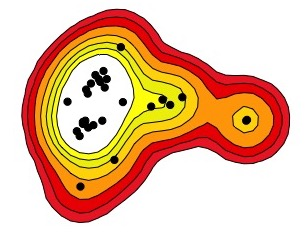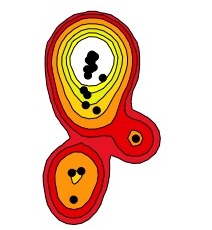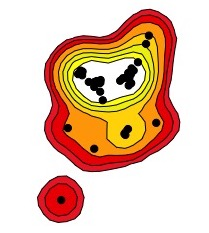Kernel density estimators, which map a utilization distribution, are one of the most popular methods for measuring home ranges. A kernel uses a function to predict how likely use is for each pixel within a grid. There are several types of kernels, such as the bivariate normal kernel and the Epanechnikov kernel. The choice of kernel is not usually that important because they typically return very similar results.

I will work with bivariate normal kernel (the default in the adehabitatHR package) on sample data to demonstrate the basics to creating kernel density estimators to measure home ranges. The code and data used are available on my GitHub page. This post builds on my previous post estimating home ranges with minimum convex polygons.

How does a kernel work? The (bivariate normal) kernel $$K$$

$K(x) = \frac{1}{2pi}exp(-\frac{1}{2}x^ix)$

is used to predict use across space ($$x$$).

$f(x) = \frac{1}{nh^2} \sum_{i=1}^{n} K\left(\frac{1}{h}(x - X_i)\right)$ where:

• n = number of relocations
• h = smoothing factor
• Xi = ith relocation of the individual

While the choice of kernel type won’t usually affect results, the choice of the smoothing factor (h) or bandwidth is important. The smoothing factor is the distance over which a data point influences the utlization distribution. A larger h results in more smoothing and increases home range size estimates.

The default of the kernelUD function in the adehabitatHR package uses the “reference bandwith”:

$h = \left(0.5 * (sd_x + sd_y)\right) * n^{−1/6}$ where:

• sdx = standard deviation of the x coordinates
• sdy = standard deviation of the y coordinates
• n = number of relocations

First, let’s load some sample data and construct kernels with the reference bandwidth.

# Read the csv file (should be in your working directory)
stringsAsFactors = FALSE)

# SpatialPointsDataFrame objects don't like missing values
# Remove rows with NA's
turtles <- turtles[!is.na(turtles$x) & !is.na(turtles$y),]

# Create a copy of the object to make into a SpatialPointsDataFrame
# Only include three columns (id, x, and y coordinates) for estimating home ranges
turtles.sp <- turtles[, c("id", "x", "y")]

# Create a SpatialPointsDataFrame by defining the coordinates
library(sp)
## Warning: package 'sp' was built under R version 3.4.4
coordinates(turtles.sp) <- c("x", "y")

# Set the coordinate reference system (CRS)
# https://www.nceas.ucsb.edu/~frazier/RSpatialGuides/OverviewCoordinateReferenceSystems.pdf
# The sample data are UTM points in WGS84 from zone 18N
proj4string(turtles.sp) <- CRS( "+proj=utm +zone=18 +datum=WGS84 +units=m +no_defs" )
library(adehabitatHR)
kernel.ref <- kernelUD(turtles.sp, h = "href")  # href = the reference bandwidth
image(kernel.ref) # plot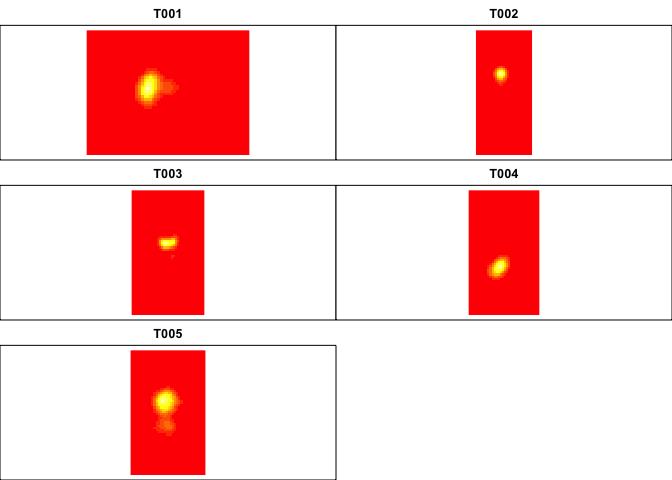kernel.ref[]@h # The smoothing factor is stored for each animal in the "h" slot
## $h ##  54.3719 ## ##$meth
##  "href"

This method of choosing h is relatively well supported by simulations and validation with telemetry data. However, it tends to estimate a larger home range than other methods of choosing h (oversmoothing).

The other most common method of choosing h is least squares cross validation. This method minimizes the error by comparing the prediction from all data points to the data minus each point.

kernel.lscv <- kernelUD(turtles.sp, h = "LSCV") # Least square cross validation
## Warning in .kernelUDs(SpatialPoints(x, proj4string = CRS(as.character(pfs1))), : The algorithm did not converge
## within the specified range of hlim: try to increase it

## Warning in .kernelUDs(SpatialPoints(x, proj4string = CRS(as.character(pfs1))), : The algorithm did not converge
## within the specified range of hlim: try to increase it
image(kernel.lscv) # plot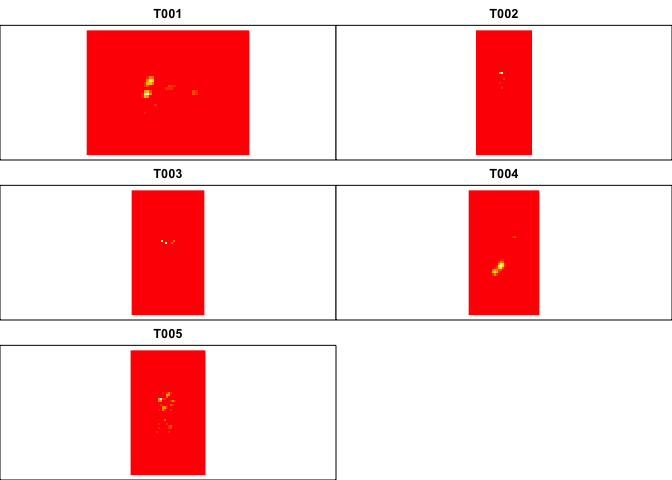We got some error messages about failure to converge, let’s see what’s going on by using the plotLSCV function. We are looking for a dip in the CV value and the h value that corresponds to the minimum CV is used. In some instances, there is no minimum (no convergence).

plotLSCV(kernel.lscv) # Look for a dip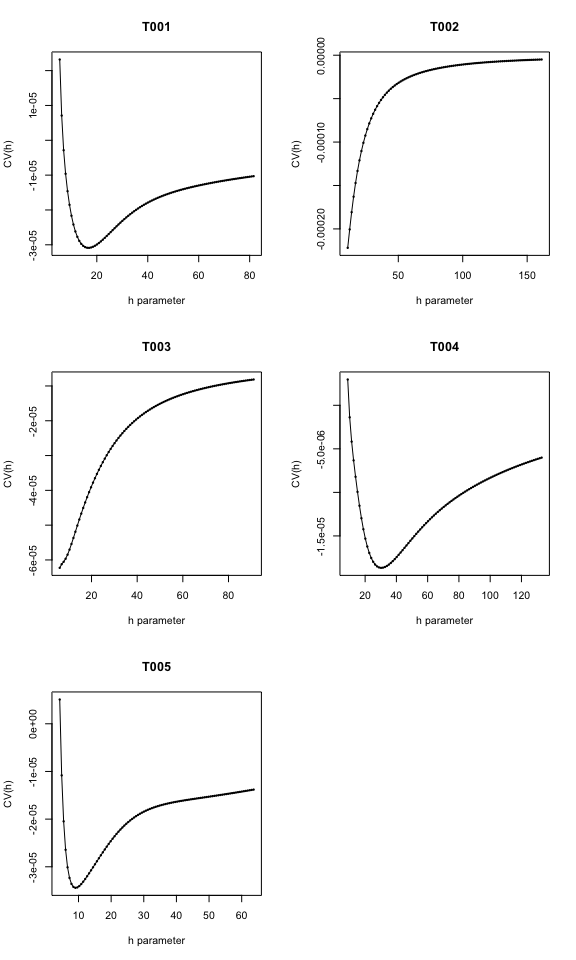You can see that there is no dip for 2/5 turtles. In fact, this issue is common when using the LSCV method of choosing h. In addition, when we look at the heat map of this kernel (above), the home ranges are heavily fragmented into many “islands.” In cases with infrequent relocation data (eg. every few days or less), I would not recommend this method of choosing h. If you have GPS collar data that collected locations very frequently, the LSCV method of selecting h may be the most appropriate. For a more in-depth discussion of this, check out this paper:

Hemson, Graham, et al. “Are kernels the mustard? Data from global positioning system (GPS) collars suggests problems for kernel home‐range analyses with least‐squares cross‐validation.” Journal of Animal Ecology 74.3 (2005): 455-463.

OK, so the obvious question is, how do I measure the size of the home range using this kernel estimator?

The easiest way to measure home ranges with kernels is to use the contour lines including a percentage of the distribution. The default of getverticeshr uses 95% contour lines (95% of estimated distribution is within the contour), but it can be manually changed. Also, the units for area depend on the input units (default of “m” in and “ha” for output). See help(getverticeshr) for details.

turtle.kernel.poly <- getverticeshr(kernel.ref, percent = 95)
print(turtle.kernel.poly)  # returns the area of each polygon
## Object of class "SpatialPolygonsDataFrame" (package sp):
##
## Number of SpatialPolygons:  5
##
## Variables measured:
##        id     area
## T001 T001 17.33054
## T002 T002 60.86678
## T003 T003 26.43825
## T004 T004 36.39425
## T005 T005 10.70571

The last thing I want to cover is how to plot kernels. Once you have created a SpatialPolygonsDataFrame with getverticeshr you can call plot on this object. Below I colour code each home range (polygon) by individual.

color <- rep("white", nrow(turtles.sp@data))
color[(turtles.sp@data$id == "T002")] <- "red" color[(turtles.sp@data$id == "T003")] <- "green"
color[(turtles.sp@data$id == "T004")] <- "blue" color[(turtles.sp@data$id == "T005")] <- "cyan"
plot(turtle.kernel.poly, col = turtle.kernel.poly@data\$id)
plot(turtles.sp, add = TRUE, col = color, pch = 21)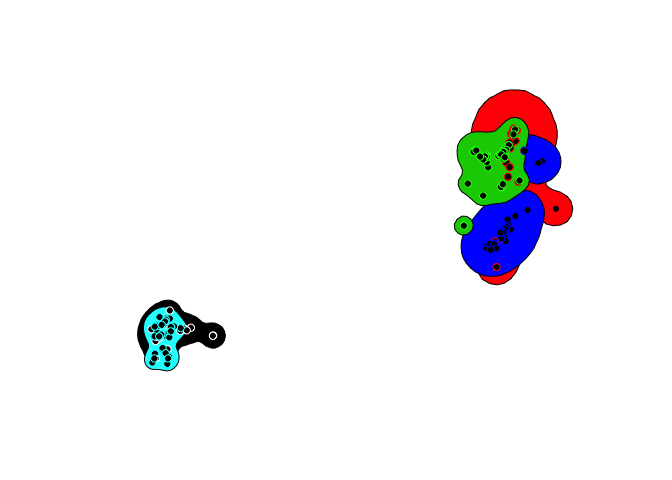Now you can easily estimate home ranges with kernel density estimators to use for mapping or analyses. You need to think carefully about the selection of h and how this affects your estimate of home range size.

Some other papers that might be helpful for thinking about home ranges:

Kie, John G., et al. “The home-range concept: are traditional estimators still relevant with modern telemetry technology?.” Philosophical Transactions of the Royal Society of London B: Biological Sciences 365.1550 (2010): 2221-2231.

Laver, Peter N., and Marcella J. Kelly. “A critical review of home range studies.” Journal of Wildlife Management 72.1 (2008): 290-298.

Harris, Stephen, et al. “Home‐range analysis using radio‐tracking data–a review of problems and techniques particularly as applied to the study of mammals.” Mammal review 20.2‐3 (1990): 97-123.

If you’re studying reptiles and amphibians, it is recommended to use an h value that creates a 95% kernel equal in area to the 100% MCP. For more details that are focussed on reptiles, see these papers:

Row, Jeffrey R., and Gabriel Blouin-Demers. “Kernels are not accurate estimators of home-range size for herpetofauna.” Copeia 2006.4 (2006): 797-802.

Bauder, Javan M., et al. “The role of the bandwidth matrix in influencing kernel home range estimates for snakes using VHF telemetry data.” Wildlife research 42.5 (2015): 437-453.